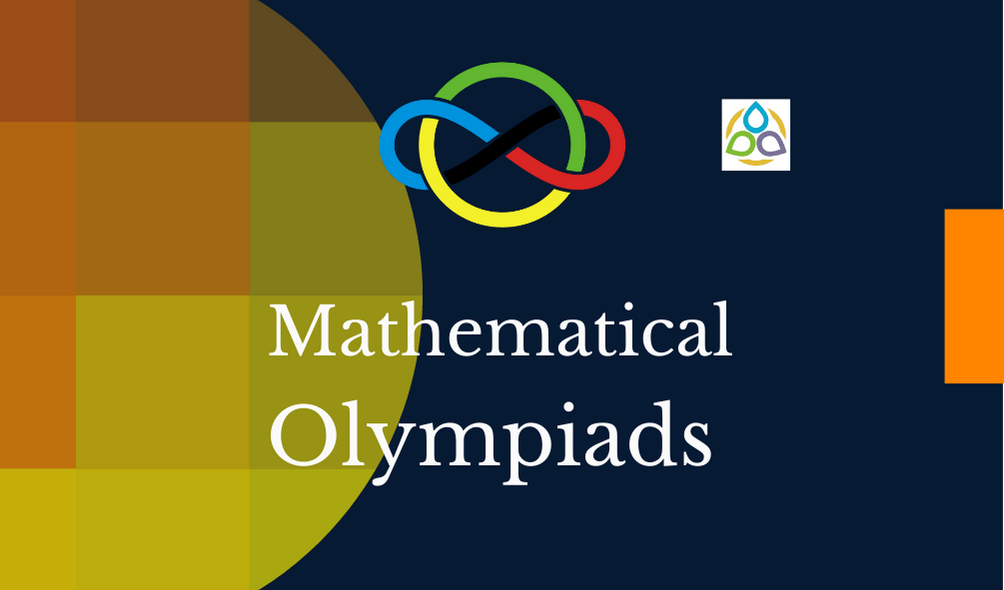# Miscellaneous Problems

Problem 68. ABC is an equilateral triangle with each side of length a. L is a line parallel to BC and b units below BC. A solid S is generated by rotating the triangle ABC about the line L; find the volume of S.

Problem 67. A hole six inches long is drilled through a sphere (the hole is six inches long after it is drilled, and the hole is through the centre of the sphere). What is the volume of the part of the sphere that remains?

Problem 66. Let n be a positive integer such that n + 1 is divisible by 12. Prove that the sum of all of the divisors of n is divisible by 12.

Problem 65. A polygon having all its angles equal and an odd number of vertices is inscribed in a circle. Prove that it must be regular.

Problem 64. Let A be a countable subset of a Euclidean plane. Show that there exists a line in the plane which contains no point of A.

Problem 63. Find the smallest natural number n that has the following properties:

(a) Its base ten representation has a 6 as the last digit;

(b) If the last digit 6, is erased and placed as the first digit in front of the remaining digits, then the resulting number is four times as large as the original number n.

Problem 62. Prove that a group G of order 15 must be cyclic.

Problem 61. Let us assume that a given pair of people either know each other or are strangers. If six people enter a room, show that there must be either three people who know each other pairwise or three people who are pairwise strangers.

Problem 60. Let T be a mapping of the Euclidean plane into itself which preserves all rational distances. Prove that T preserves all distances.

Problem 59. Call a number P fortunate, if it can be written with four equal digits in some base b Î N.

(a) Clearly 2222 is fortunate; why is 2000 fortunate?

(b) Find the greatest fortunate number less than 2000.

Problem 58. Each side of a square is subdivided into 101 segments. Find the number of different triangles that have their vertices at these 400 points of subdivision.

Problem 57. Given n + 1 integers between 1 and 2n inclusive, prove that one of them must be a multiple of some other one.

Problem 56. N points are chosen on a circle so that when all segments are drawn between all pairs of points, no three segments intersect at the same point. In how many points do these segments intersect in the interior of the circle?

Problem 55. A positive integer n is called composite if there are positive integers p ≠ 1 and q ≠ 1 so that n = pq. Find a sequence of 10 consecutive positive integers each of which is composite and less than 1,000,000.

Problem 54. Find all positive integers which are one more than the sum of the squares of their base ten digits. For example, 35 = 1 + 32 + 52.

Problem 53. If a, b and c are integers and if a + b + c is even, find an integer n (in terms of a, b and e) such that ab + n, bc + n and ca + n are all squares of integers.

Problem 52. Let P be any point inside an equilateral triangle T. Show that the sum of the three distances from P to the sides of T is constant.

Problem 51. What is the probability that a dart, hitting a square board at random, lands nearer the centre than the edge?

Problem 50. Find the largest possible area of a pentagon with five sides of length 1 and a right interior angle.

Problem 49. Find all solutions (x, y) of the equation xy = yx for real numbers x, y > 0.

Problem 48. Find all integers A, B, C, D, E (ABCDE) which, when added in pairs, yield only the sums 401, 546, 691, and 836.

Problem 47. The sides of a triangle have lengths 4, 5, and 6. Show that one of its angles is twice another.

Problem 46. Find all sets of five positive integers whose sum equals their product.

Problem 45. Show that if one of the coordinates of the centre of a circle is irrational, then the circle contains at most two points both of whose coordinates are rational.

1.DEB JYOTI MITRA says:
1.Math1089 says: